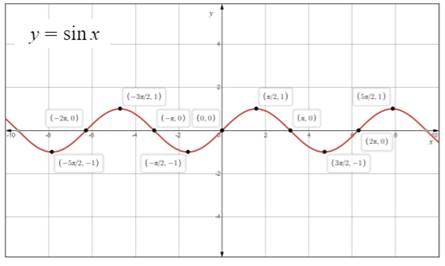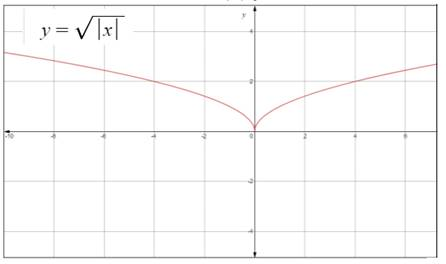# the relation between thegiven graph of y = f | x | and graph of f .### Single Variable Calculus: Concepts...

4th Edition
James Stewart
Publisher: Cengage Learning
ISBN: 9781337687805### Single Variable Calculus: Concepts...

4th Edition
James Stewart
Publisher: Cengage Learning
ISBN: 9781337687805

#### Solutions

Chapter 1.3, Problem 27E

a.

To determine

## To find:the relation between thegiven graph of y=f|x| and graph of f .

Expert Solution

1. Wipe the part of graph which is to the left of y -axis.
2. Now reflect the right part of graph in y -axis.

### Explanation of Solution

Given:

The relation between the given graph of y=f|x| and graph of f .

Calculation:

As it isknown that

|x|={xfor x0xfor x<0 .

Therefore,

f|x|={f(x)for x0f(x)for x<0

Set the graph of y=f|x| and graph of y=f(x) into two steps:

1. Wipe the part of graph which is to the left of y -axis.
2. Now reflect the right part of graph iny -axis.

b.

To determine

Expert Solution

### Explanation of Solution

Given:

Graph of y=sinx .

Calculation:

Graph of y=sinx :c.

To determine

Expert Solution

### Explanation of Solution

Given:

The graph of y=|x| .

Calculation:

Graph of y=|x| :### Have a homework question?

Subscribe to bartleby learn! Ask subject matter experts 30 homework questions each month. Plus, you’ll have access to millions of step-by-step textbook answers!